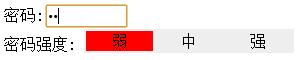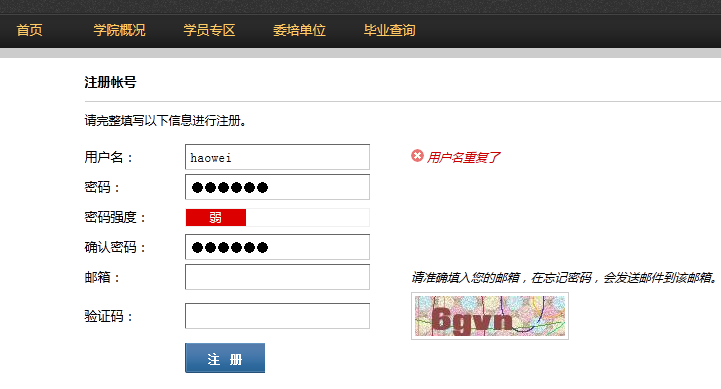# js密码强度检测_javascript技巧

2020年1月18日19:46:10```

JS判断密码强度

//判断输入密码的类型
function CharMode(iN){
if (iN>=48 && iN <=57) //数字
return 1;
if (iN>=65 && iN <=90) //大写
return 2;
if (iN>=97 && iN <=122) //小写
return 4;
else
return 8;
}
//bitTotal函数
//计算密码模式
function bitTotal(num){
modes=0;
for (i=0;i<4;i++){
if (num & 1) modes++;
num>>>=1;
}
return modes;
}
//返回强度级别
function checkStrong(sPW){
if (sPW.length<=4)
return 0; //密码太短
Modes=0;
for (i=0;i<sPW.length;i++){
//密码模式
Modes|=CharMode(sPW.charCodeAt(i));
}
return bitTotal(Modes);
}

//显示颜色
function pwStrength(pwd){
O_color="#eeeeee";
L_color="#FF0000";
M_color="#FF9900";
H_color="#33CC00";
if (pwd==null||pwd==''){
Lcolor=Mcolor=Hcolor=O_color;
}
else{
S_level=checkStrong(pwd);
switch(S_level) {
case 0:
Lcolor=Mcolor=Hcolor=O_color;
case 1:
Lcolor=L_color;
Mcolor=Hcolor=O_color;
break;
case 2:
Lcolor=Mcolor=M_color;
Hcolor=O_color;
break;
default:
Lcolor=Mcolor=Hcolor=H_color;
}
}
document.getElementById("strength_L").style.background=Lcolor;
document.getElementById("strength_M").style.background=Mcolor;
document.getElementById("strength_H").style.background=Hcolor;
return;
}

密码强度:

弱
中
强

``````
function AuthPasswd(string) {
if(string.length >=6) {
if(/[a-zA-Z]+/.test(string) && /[0-9]+/.test(string) && /\W+\D+/.test(string)) {
noticeAssign(1);
}else if(/[a-zA-Z]+/.test(string) || /[0-9]+/.test(string) || /\W+\D+/.test(string)) {
if(/[a-zA-Z]+/.test(string) && /[0-9]+/.test(string)) {
noticeAssign(-1);
}else if(/\[a-zA-Z]+/.test(string) && /\W+\D+/.test(string)) {
noticeAssign(-1);
}else if(/[0-9]+/.test(string) && /\W+\D+/.test(string)) {
noticeAssign(-1);
}else{
noticeAssign(0);
}
}
}else{
noticeAssign(null);
}
}

function noticeAssign(num) {
if(num == 1) {
\$('#weak').css({backgroundColor:'#009900'});
\$('#middle').css({backgroundColor:'#009900'});
\$('#strength').css({backgroundColor:'#009900'});
\$('#strength').html('很强');
\$('#middle').html('');
\$('#weak').html('');
}else if(num == -1){
\$('#weak').css({backgroundColor:'#ffcc33'});
\$('#middle').css({backgroundColor:'#ffcc33'});
\$('#strength').css({backgroundColor:''});
\$('#weak').html('');
\$('#middle').html('中');
\$('#strength').html('');
}else if(num ==0) {
\$('#weak').css({backgroundColor:'#dd0000'});
\$('#middle').css({backgroundColor:''});
\$('#strength').css({backgroundColor:''});
\$('#weak').html('弱');
\$('#middle').html('');
\$('#strength').html('');
}else{
\$('#weak').html(' ');
\$('#middle').html(' ');
\$('#strength').html(' ');
\$('#weak').css({backgroundColor:''});
\$('#middle').css({backgroundColor:''});
\$('#strength').css({backgroundColor:''});
}
}

```• 本文由 发表于 2020年1月18日19:46:10
• 转载请务必保留本文链接：https://www.qieseo.com/410059.html